### 道奇(进口) 酷威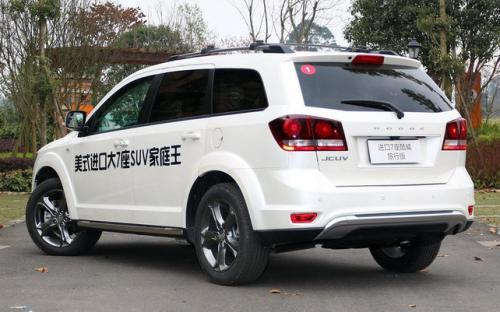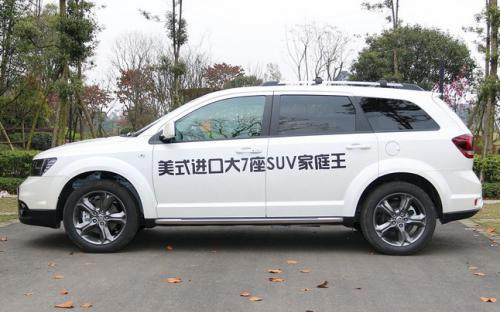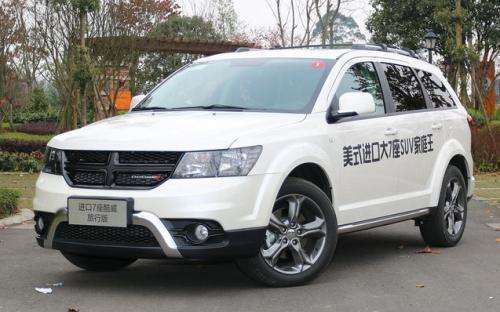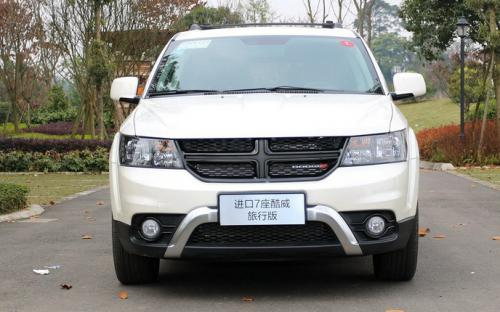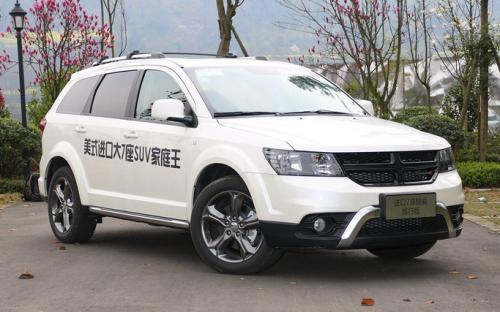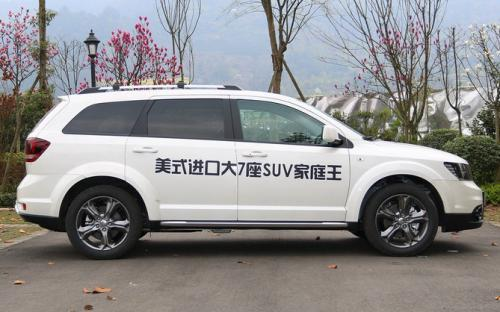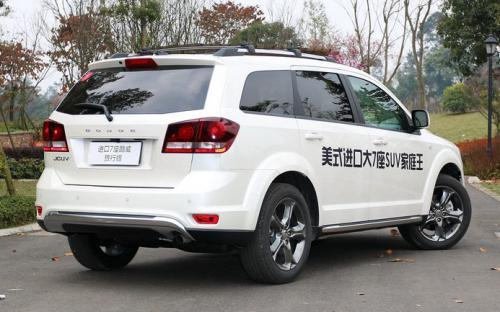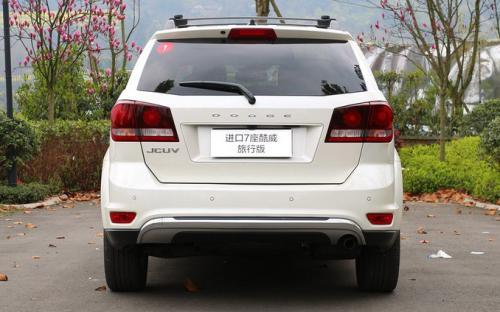5 种颜色可选2016款最低售价：29.99 万元起

4910(mm)1878(mm)1751(mm)##### 配置亮点：
• 胎压监测装置

• ISOFIX儿童座椅接口

• 车身稳定控制(ESC/ESP/DSC等)

• 电动天窗

• 定速巡航

• 后倒车雷达

• 真皮座椅

• GPS导航系统

• 氙气大灯

• 后视镜加热

• 提交
2016款 2.4L 两驱旅行版 (111张)

道奇(进口) 酷威 绕车实拍• 道奇(进口) 酷威 在售车型

排量 车型 厂商指导价 本地最低报价 购车工具
2.4L
2.4L 两驱旅行版 6挡手自一体
29.99万
29.99万

2.0T
2.0TD 四驱旅行版 柴油 6挡手自一体
29.99万
29.99万

道奇(进口) 酷威 经销商

查看更多 >>

### 道奇(进口) 酷威 动力加速

酷威 0-100公里加速时间分布在 0.0-秒 属于 超跑级

动力级别 加速时间 车型

道奇(进口) 酷威 视频

道奇(进口) 酷威 新闻资讯

# 新款道奇酷威旅行版上市 售29.99万元

进口新车 超过8751次关注

2016年3月11日，2016款道奇酷威旅行版车型正式在国内上市,提供了2.4L（汽油）和2.0T（柴油）两种排量共2款车型，售价均为29.99万元。

即将上市 超过7513次关注

# 酷威2.0TD四驱柴油版奇袭郑州

试驾评测 超过8981次关注

“4T男神”全新进口酷威2.0TD四驱柴油版郑州区域上市试驾会，小编现场体验四驱系统对各种路况的超强适应性，以及2.0TD柴油发动机的强劲动力！

评测 超过4120次关注

旅行版车型相比在售的2013款车型来说，在外观上和配置方面有了一定程度的调整，使得它显现出更多的运动感和精致感。首先它的标志性十字进气格栅采用了黑色设计，和...

# 30万以下7座SUV推荐 大空间家用便利

导购 超过2390次关注

酷威是30万以下少有的进口7座SUV车型，搭载2.4L或3.6L发动机，搭配6挡手自一体变速箱，其中2.4L有两款车型皆为两驱的型号，而3.6L只有一款车型，采用四驱的驱动方式...

# 菲亚特菲跃/酷威对比实拍 比酷威更现代

导购 超过3763次关注

纵观全球汽车市场，已经有越来越多的车型采用完全相同的底盘，甚至搭载完全相同的动力系统，却挂着不同品牌的商标，有人把这样的车型称作“孪生兄弟”。现在，菲亚...

# 酷威旅行版亮相2014成都车展 售29.99万

新闻 超过2243次关注

2014成都车展已经正式开幕，本届车展上，道奇酷威旅行版车型正式上市。新车的售价为29.99万。

新闻 超过2232次关注

新闻 超过2446次关注

猜你喜欢

﻿
• 快速找车
• 选择品牌
• 选择品牌
• A  奥迪
• A  阿斯顿·马丁
• A  阿尔法·罗密欧
• B  宝沃
• B  布加迪
• B  巴博斯
• B  保时捷
• B  宾利
• B  奔驰
• B  宝马
• B  本田
• B  别克
• B  标致
• B  比亚迪
• B  宝骏
• B  北汽制造
• B  北汽新能源
• B  北汽幻速
• B  北汽威旺
• B  北京汽车
• B  奔腾
• B  北汽绅宝
• C  长安
• C  长安商用
• C  长城
• C  昌河
• D  大众
• D  道奇
• D  DS
• D  东南
• D  东风风神
• D  东风风行
• D  东风小康
• D  东风风度
• D  东风
• F  福特
• F  丰田
• F  菲亚特
• F  法拉利
• F  福田
• F  福迪
• F  福汽启腾
• G  观致
• G  广汽传祺
• G  广汽吉奥
• G  GMC
• H  红旗
• H  汉腾汽车
• H  哈弗
• H  哈飞
• H  海格
• H  海马
• H  华颂
• H  黄海
• H  华泰
• H  恒天
• J  吉利汽车
• J  捷豹
• J  Jeep
• J  江淮
• J  江铃
• J  金杯
• J  九龙
• J  金旅
• K  凯翼
• K  凯迪拉克
• K  克莱斯勒
• K  科尼塞克
• K  卡威
• K  开瑞
• L  路虎
• L  林肯
• L  劳斯莱斯
• L  兰博基尼
• L  雷克萨斯
• L  铃木
• L  雷诺
• L  理念
• L  力帆
• L  莲花汽车
• L  猎豹
• L  路特斯
• L  陆风
• M  马自达
• M  MG
• M  MINI
• M  玛莎拉蒂
• M  摩根
• M  迈凯轮
• N  纳智捷
• O  欧宝
• O  讴歌
• O  欧朗
• Q  奇瑞
• Q  起亚
• Q  启辰
• R  日产
• R  荣威
• R  瑞麒
• S  三菱
• S  斯威汽车
• S  萨博
• S  smart
• S  斯柯达
• S  斯巴鲁
• S  思铭
• S  双龙
• S  上汽大通
• S  双环
• T  特斯拉
• T  腾势
• W  沃尔沃
• W  五菱汽车
• W  五十铃
• W  威兹曼
• W  威麟
• X  现代
• X  雪佛兰
• X  雪铁龙
• X  西雅特
• Y  一汽
• Y  英菲尼迪
• Y  英致
• Y  依维柯
• Y  野马汽车
• Y  永源
• Z  众泰
• Z  中华
• Z  中兴
• Z  知豆
• 选择车系
• 选择车系
• 车型对比
• 选择品牌
• 选择品牌
• A  奥迪
• A  阿斯顿·马丁
• A  阿尔法·罗密欧
• B  宝沃
• B  布加迪
• B  巴博斯
• B  保时捷
• B  宾利
• B  奔驰
• B  宝马
• B  本田
• B  别克
• B  标致
• B  比亚迪
• B  宝骏
• B  北汽制造
• B  北汽新能源
• B  北汽幻速
• B  北汽威旺
• B  北京汽车
• B  奔腾
• B  北汽绅宝
• C  长安
• C  长安商用
• C  长城
• C  昌河
• D  大众
• D  道奇
• D  DS
• D  东南
• D  东风风神
• D  东风风行
• D  东风小康
• D  东风风度
• D  东风
• F  福特
• F  丰田
• F  菲亚特
• F  法拉利
• F  福田
• F  福迪
• F  福汽启腾
• G  观致
• G  广汽传祺
• G  广汽吉奥
• G  GMC
• H  红旗
• H  汉腾汽车
• H  哈弗
• H  哈飞
• H  海格
• H  海马
• H  华颂
• H  黄海
• H  华泰
• H  恒天
• J  吉利汽车
• J  捷豹
• J  Jeep
• J  江淮
• J  江铃
• J  金杯
• J  九龙
• J  金旅
• K  凯翼
• K  凯迪拉克
• K  克莱斯勒
• K  科尼塞克
• K  卡威
• K  开瑞
• L  路虎
• L  林肯
• L  劳斯莱斯
• L  兰博基尼
• L  雷克萨斯
• L  铃木
• L  雷诺
• L  理念
• L  力帆
• L  莲花汽车
• L  猎豹
• L  路特斯
• L  陆风
• M  马自达
• M  MG
• M  MINI
• M  玛莎拉蒂
• M  摩根
• M  迈凯轮
• N  纳智捷
• O  欧宝
• O  讴歌
• O  欧朗
• Q  奇瑞
• Q  起亚
• Q  启辰
• R  日产
• R  荣威
• R  瑞麒
• S  三菱
• S  斯威汽车
• S  萨博
• S  smart
• S  斯柯达
• S  斯巴鲁
• S  思铭
• S  双龙
• S  上汽大通
• S  双环
• T  特斯拉
• T  腾势
• W  沃尔沃
• W  五菱汽车
• W  五十铃
• W  威兹曼
• W  威麟
• X  现代
• X  雪佛兰
• X  雪铁龙
• X  西雅特
• Y  一汽
• Y  英菲尼迪
• Y  英致
• Y  依维柯
• Y  野马汽车
• Y  永源
• Z  众泰
• Z  中华
• Z  中兴
• Z  知豆
• 选择车系
• 选择车系
• 选择车型
• 选择车型
• 意见反馈